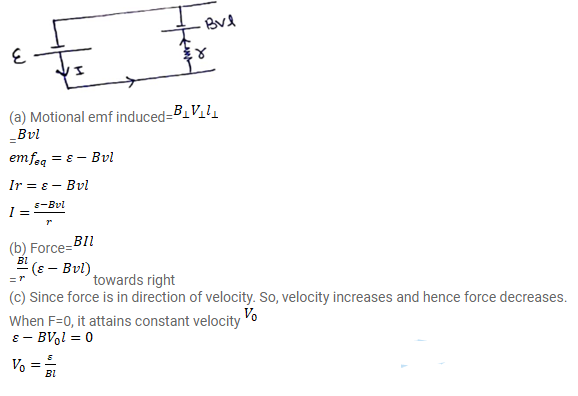# Figure shows a smooth pair of thick metallic rails connected

Question:

Figure shows a smooth pair of thick metallic rails connected across a battery of emf $\varepsilon$ having a negligible internal resistance. A wire ab of length I and resistance $\mathrm{r}$ can slide smoothly on the rails. The entire system lies in a horizontal plane and is immersed in a uniform vertical magnetic field $B$. At and instant $t$, the wire is given a small velocity $v$ towards right.

(a) Find the current in it at this instant. What is the direction of the current?

(b) What is the force acting on the wire at this instant?

(c) Show that after some time the wire ab will slide with a constant velocity. Find this velocity.

Solution: